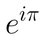# Where was I born? (with respect to the sun)

I think it is quite fun to think of the birthday as a time where you are closest to the place in which you were born. This, of course, makes the actual date of your birthday irrelevant, as you have to take leap years and the $6$ hours desynchronization thing into account, but it is still very easy to calculate, just add $6$ hours to your time of birth every non leab year, and subtract $18$ every leap year to figure out, with much more precision, this moment of closest approach.

However, something much more challenging, and much more interesing, in my opinion, is figuring out exactly where you were born, and calculating in what direction, and how far that where is in the moment of your closest approach birthday, just so you can impress your friends by pointing at a direction and saying "Look! I was born there, about $5$ Earth diameters away"

My question is: What do you have to take into consideration to get a reasonable degree of precision, say $15º$ or less in the direction you need to point? Is it enough to just assume the earth is arround the same position and just see how much the rotation is different? Do you have to account for the precession of the Earth's orbit? And how about minor effects like Milankovich cycles?Note by Pedro Cardoso
2 years, 5 months ago

This discussion board is a place to discuss our Daily Challenges and the math and science related to those challenges. Explanations are more than just a solution — they should explain the steps and thinking strategies that you used to obtain the solution. Comments should further the discussion of math and science.

When posting on Brilliant:

• Use the emojis to react to an explanation, whether you're congratulating a job well done , or just really confused .
• Ask specific questions about the challenge or the steps in somebody's explanation. Well-posed questions can add a lot to the discussion, but posting "I don't understand!" doesn't help anyone.
• Try to contribute something new to the discussion, whether it is an extension, generalization or other idea related to the challenge.

MarkdownAppears as
*italics* or _italics_ italics
**bold** or __bold__ bold
- bulleted- list
• bulleted
• list
1. numbered2. list
1. numbered
2. list
Note: you must add a full line of space before and after lists for them to show up correctly
paragraph 1paragraph 2

paragraph 1

paragraph 2

[example link](https://brilliant.org)example link
> This is a quote
This is a quote
    # I indented these lines
# 4 spaces, and now they show
# up as a code block.

print "hello world"
# I indented these lines
# 4 spaces, and now they show
# up as a code block.

print "hello world"
MathAppears as
Remember to wrap math in $$ ... $$ or $ ... $ to ensure proper formatting.
2 \times 3 $2 \times 3$
2^{34} $2^{34}$
a_{i-1} $a_{i-1}$
\frac{2}{3} $\frac{2}{3}$
\sqrt{2} $\sqrt{2}$
\sum_{i=1}^3 $\sum_{i=1}^3$
\sin \theta $\sin \theta$
\boxed{123} $\boxed{123}$